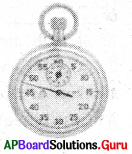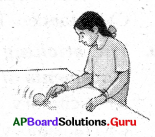Practice the AP 7th Class Science Bits with Answers Chapter 4 Motion and Time on a regular basis so that you can attempt exams with utmost confidence.

## AP State Syllabus 7th Class Science Bits 4th Lesson Motion and Time with Answers

Question 1.
Motion and rest are
A) absolute
B) fixed
C) relative
D) None
C) relative

Question 2.
The girl on the bicycle is in in relation to the road.
A) rest
B) motion
C) oscillatory motion
D) rotatory motion
B) motionQuestion 3.
Motion of a mango falling from a tree is
A) oscillatory motion
B) translatory motion
C) at rest
D) rotatory motion
B) translatory motion

Question 4.
Ringing of a bell is an example for
A) Rotatory motion
B) Translatory motion
C) Rectilinear motion
D) Oscillatory motion
D) Oscillatory motion

Question 5.
A spinning top has
A) Translatory motion
B) Rotatory motion
C) Oscillatory motion
D) Rectilinear motion
B) Rotatory motion

Question 6.
1 km/h = ………… m/s.
A) 18/5
B) 15/8
C) 5/18
D) 15/18
C) 5/18Question 7.
This goes fast
A) Air plane
B) Train
C) Bus
D) Car
A) Air plane

Question 8.
Speedometer is not fixed for this.
A) Car
B) Scooter
C) Bus
D) Bicycle
D) Bicycle

Question 9.
In uniform motion the distance is
A) Equal
B) Unequal
C) Zero
D) Maximum
A) Equal

Question 10.
In non-uniform motion the body covers distances.
A) Equal
B) Unequal
C) Constant
D) Circular
B) UnequalQuestion 11.
Movement of housefly shows ………… motion.
A) Non – uniform
B) Rotatory
C) Linear
D) translatory
D) translatory

Question 12.
Motion of needle in a running sewing machine shows
A) Oscillatory
B) Circular
C) Rotatory
D) All of these
A) Oscillatory

Question 13.
Soldiers marching in a parade is
A) Straight line
B) Curved line
C) Oscillatory
D) Rotatory
A) Straight line

Question 14.
Motion of the ball in pin board
A) Curvilinear
B) Rectilinear
C) Both A & B
D) Oscillatory
C) Both A & BQuestion 15.
A body moves in a fixed path and centre is called
A) Translatory
B) Rotatory
C) Circular
D) Oscillatory
B) Rotatory

Question 16.
Motion of wheel of the sewing machine
A) Rotatory
B) Translatory
C) Linear
D) Oscillatory
A) Rotatory

Question 17.
The basic unit of time is
A) Month
B) Second
C) Hour
D) Days
B) Second

Question 18.
The speed is ………
A) Distance/time
B) Time/distance
C) Distance x Time
D) None of these
A) Distance/timeQuestion 19.
The sticking machine shows the movements of
A) Oscillatory
B) Rotatory
C) Translatory
D) All
D) All

Question 20.
In a sewing machine motion of cloth is
A) Linear
B) Rotatory
C) Circular
D) Oscillatory
A) Linear

Question 21.
Units for measuring time
A) Days
B) Weeks
C) Hours
D) All of these
D) All of these

Question 22.
Speedometer shows the distance travelled in
A) kilometers
B) meters
C) km/s
D) m/s
A) kilometersQuestion 23.
The needle of the speedometer explains
A) Velocity
B) Speed
C) Distance
D) Acceleration
B) Speed

Question 24.
Name the instrument for measuring distance
A) Odometer
B) Speedometer
C) Altimeter
D) All of these
A) Odometer

Question 25.
Total distance travelled / total time indicates
A) Speed
B) Velocity
C) Power
D) Average speed
D) Average speed

Question 26.
Hand of clock, tape of tape recorder examples for motion.
A) Oscillatory
B) Translatory
C) Linear
D) Rotatory
D) RotatoryQuestion 27.
The correct rela tion between speed(v) distance(s) and time(t) is
A) v = s / t
B) v = t/s
C) v = s/2t
D) v = s x t
A) v = s / t

Question 28.
One of the following is not showing oscillatory motion
A) Pendulum
B) Sewing needle
D) Kite
D) Kite

Question 29.
If a body covers equal distance in equal intervals it is
A) Rotatory
B) Uniform motion
C) Non-uniform motion
D) Oscilatory
B) Uniform motion

Question 30.
If a body covers an equal distance in equal intervals of time it is
A) Uniform motion
B) Non-uniform motion
C) Rotatory motion
D) Oscilatory motion
B) Non-uniform motionQuestion 31.
Flying of kite in air shows
A) Non-uniform motion
B) Uniform motion
C) Rotatory motion
D) Oscilatory
A) Non-uniform motion

Question 32.
Blades of ceiling fan show the motion of
A) Translatory
B) Oscilatory
C) Rotatory
D) Oscilatory
C) Rotatory

Question 33.
If the translatory motion moves long straight line, it is
A) Rectilinear motion
B) Curvilinear motion
C) Rotatory motion
D) Oscilatory
A) Rectilinear motion

Question 34.
Translatory motion moves long a curved path it is
A) Rectilinear motion
B) Curvilinear motion
C) Rotatory motion
D) Oscilatory motion
B) Curvilinear motionQuestion 35.
Movement of train on tracks is called
A) Rectilinear
B) Curvilinear
C) Rotatory
D) Oscilatory
A) Rectilinear

Question 36.
Movement of needle in car speedometer is
A) Rectilinear
B) Curvilnear
C) Rotatory
D) Oscilatory
B) Curvilnear

Question 37.
Motions of coins on a carrom board is
A) Rectilinear
B) Curvilnear
C) Both A and B
D) None
C) Both A and B

Question 38.
Motion of earth around the Sun is
A) Translatory
B) Rotatory
C) Oscilatory
D) Both A and B
D) Both A and BQuestion 39.
A bouncing ball movement is
A) Rotatory
B) Curvilinear
C) Oscilatory
D) Rectilinear
C) Oscilatory

Question 40.
Month, Season, Ayanam are the units for
A) Time
B) Speed
C) Mass
D) Weight
A) Time

Question 41.
The basic unit of time is
A) Day
B) Hour
C) Second
D) Minute
C) Second

Question 42.
Unit of speed in S.I system is
A) Meter/second
B) C.M/s
C) M/H
D) All
A) Meter/secondQuestion 43.
Name the instrument shown in the rigA) Barometer
B) Speedometer
C) Altimeter
D) Thermometer
B) Speedometer

Question 44.
Name the instrument shown in the rigA) Watch
B) Stopwatch
C) Speedometer
D) Alaram
B) Stopwatch

Question 45.
The motion shown in the figure isA) Rotatory
B) Oscilatory
C) Translatory
D) Curveior
B) Oscilatory

Question 46.
Motion of a drill bit have
A) Translatory
B) Rotatory
C) Both A and B
D) Oscilatory
C) Both A and BQuestion 47.
In the figure the ball shows the movements ofA) Rotatory
B) Translatory
C) Oscilatory
D) Both A and B
D) Both A and B

Question 48.
The movement of needle in the picture isA) Rectilinear motion
B) Circulatory motion
C) Rotatory motion
D) Oscillatory motion
D) Oscillatory motion

Question 49.
Motion of air cooler fan
A) Oscillatory motion
B) Rotatory motion
C) Translatory motion
D) Curvilinear motion
B) Rotatory motion

Question 50.
Choose the right one.
i) A train runs from Visakhapatnam to Vijayawada. It covers distance of 420 km in 7hrs and next distance of 60 km in one hour.
ii) Gopi takes part in a car race. He drives a distance of 70 km each in time first, second and third hours. Which of the following statements is true?
A) i – is an example uniform motion and ii – is an example of non-uniform motion
B) i – is an example of non-uniform motion and ii – is an example of uniform motion
C) i and ii are examples of uniform motions
D) i and ii are examples of non-uniform motions
D) i and ii are examples of non-uniform motionsQuestion 51.
Which one is right hypothesis?
A) Movements of hands in watch is translatory motion.
B) Motion of a ringing bell is rotatory motion.
C) Motion in top is linear motion.
D) Movement of train on tracks is translatory motion.
D) Movement of train on tracks is translatory motion.

Question 52.
John tied a stone to a string and whirled it around. What type of motion do you find in it?
A) Translatory motion
B) Oscillatory motion
C) Rotatory motion
D) Linear motion
A) Translatory motion

Question 53.
Motion of an arrow from a bow is an example of …………
A) Translatory motion
B) Rotatory motion
C) Periodic motion
D) Oscillatory motion
A) Translatory motion

Question 54.
Motion of the Earth around the Sunnis an example of ………..
A) Translatory motion
B) Periodic motion
C) Rotatory motion
D) Oscillatory motion
B) Periodic motionQuestion 55.
This type of motion is exhibited by the motion of a girl swinging on a
A) Translatory motion
B) Rotatory motion
C) Oscillatory motion
D) Periodic motion